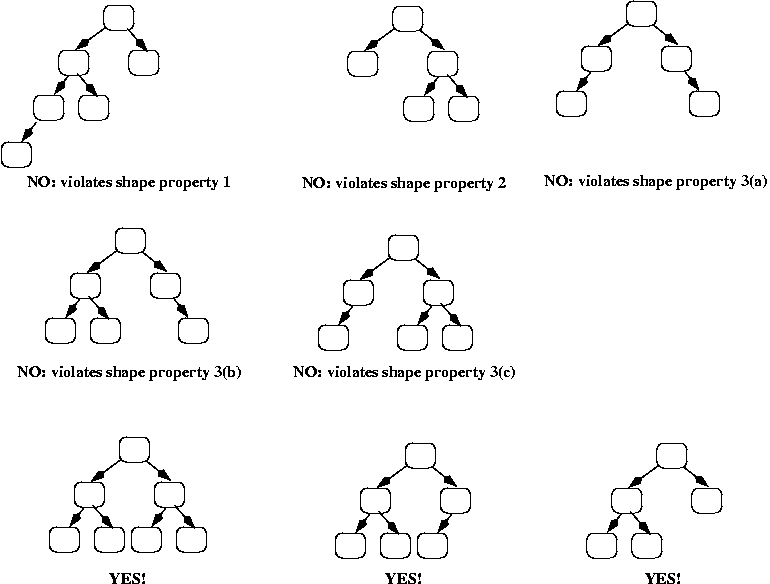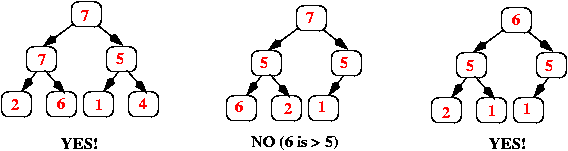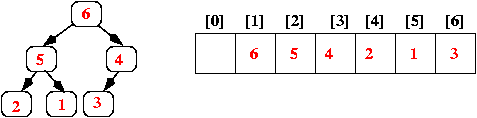## Hash Table

### Collision resolution:

• $O(N)$ worst case
• most common approach

#### 2. Chaining with BST

• $O(logN)$ worst case
• take more space than linked list
• hence, rarely used unless we had extremely nonuniform distribution

#### 3. Open Addressing with Linear Probing

• idea: move to next cloest closed open spot
• no bucket chaining, total entries limited by array size
• issue of clustering because of linear probing
• worst case query time get() is $O(N)$

#### 4. Quadratic Probing and Double Hashing

• instead of probing linear as in approach 3, we prob quadratically
• or use second hash function to random determine prob distance (reduce chance of clustering)

## Trie

### Why Trie?

• hashing search, insert, delete takes $O(L)$ (for hash() opeartion) in average, but need to handle collision
• Trie: insert and serach string in $O(L)$, where $L$ is the length of string, no need to handle collision
• prefix search & autocomplete

### Implementation1: Array

• fixed sized array, limited to lower case letters
• use c - 'a' to index array for character c
• waste of unused space

example:

### Implementation2: HashMap

• slower query/search than array (time for hash(), etc)
• saves spaces, only store the children nodes we need
• more flexible in functionality (suppoert upper-cases letter, or TrieNode could store words in hiericy, Quantcast OA JSON storage & query)

## Binary Search Tree (BST)

Test program:

ref:

http://blog.csdn.net/lemon_tree12138/article/details/50366993

http://blog.csdn.net/wanghao109/article/details/13508357

http://www.cnblogs.com/gaochundong/p/binary_search_tree.html

http://www.cnblogs.com/gaochundong/archive/2013/04/24/csharp_binary_tree.html

## Stack

### Using Array

• works, however, array has fixed size, space used NOT proportional to number of items in list.
• require expansion $O(n)$ extra time complexity for each expandsion, but $O(1)$ amortized/average time
• OR better always $O(1)$ for push() w/ a shadow array.

• dynamically size, space used proportional to number of items in list, no expansion required.
• extra space to store next pointer for each of the value (Array no such extra pointer)

Test Program:

## Queue

• i used LinkedList for implementation
• insert to tail, remove from head (difference to Stack)

Test Program:

## Heap

### Heap Property

• Heap is a tree-based datastructure with two special properties:
• ORDER property
• SHAPE property
• A common implementation of a heap is the binary heap, in which the tree is a binary tree (complete binary tree)
• Hence we could use array as representation of a heap
• All examples given in this section are max-heap, min-heap is similiar

The ORDER property:

For every node n, the value in n is greater than or equal to the values in its children (and thus is also greater than or equal to all of the values in its subtrees).

The SHAPE property:

• All leaves are either at depth d or d-1 (for some value d).
• All of the leaves at depth d-1 are to the right of the leaves at depth d.
• There is at most 1 node with just 1 child, that child is the left child of its parent, and it is the rightmost leaf at depth.

The SHAPE property Examples:The ORDER property Examples:### Implementing priority queues using heaps

• Array or ArrayList
• Starts at position 1 (instead of 0)
• The root of the heap is always in array.
• In general, if a node is in array[k], then its left child is in array[k*2], and its right child is in array[k*2 + 1].
• If a node is in array[k], then its parent is in array[k/2] (using integer division, so that if k is odd, then the result is truncated; e.g., 3/2 = 1).### Insert() operation

• insert to the end of the array (the right most leave to tree)
• swap with its parent if it is larger than its parent value
• recursively perform step two until hit root OR step 2 fails

### removeMax() operation

• swap root with last element in array, and pop the last element
• swap new root with its larger childern
• recursively perform step two util its fails

## Graph

Graph consisits of:

• finite set of vertices called nodes
• finite set of vertice pair $(u, v)$ called edges
• two types of graph: directed graph and undirected graph
• undirected graph has symmetric adjacency matrix

[TODO] X-code PPT
https://goo.gl/pd5XDK
https://goo.gl/hciJzA

updated: 05/30/2018